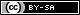# Dev Blog

 ./dev Original heme by orderedlist (CC-BY-SA) Where applicable, all content is licensed under a CC-BY-SA.# PCB Notes

Copper thickness is commonly expressed in oz, for example 1 oz Cu. This is shorthand for the height of copper if spread over a square foot surface.

To calculate the height for 1 oz Cu, we need a few conversions:

• $1\ \mathrm{oz} = 0.0625\ \mathrm{lbs}$
• $\mathrm{Cu}\ \mathrm{density} = 8.96 \frac{\mathrm{g}}{\mathrm{cm}^3}$
• $1\ \mathrm{g} \approx 0.00220462\ \mathrm{lbs}$
• $1\ \mathrm{cm} \approx 0.393701\ \mathrm{in}$

This implies:

• $\mathrm{Cu}\ \mathrm{density} = \frac{8.96 \cdot 0.00220462\ \mathrm{lbs}}{(0.393701^3)\ \mathrm{in}^3} \approx 0.324 \frac{\mathrm{lbs}}{\mathrm{in}^3}$
• $h\ \mathrm{in} = \frac{1\ \mathrm{oz}}{ 1\ \mathrm{ft}^2 } = \frac{0.0625\ \mathrm{lbs}}{ 144\ \mathrm{in}^2 } \approx .00134$

Or, in general,

$$z\ \mathrm{oz}\ \mathrm{Cu} \rightarrow z \cdot 1.34\ \mathrm{mil}$$

Where $1\ \mathrm{mil} = \frac{1}{1000}\ \mathrm{in}$.